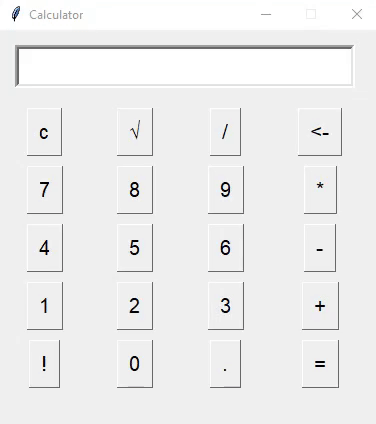GeeksforGeeks App
Open AppBrowser
Continue

# Python – Dynamic GUI Calculator using Tkinter module

Python provides many options for developing GUI like Kivy, PyQT, WxPython, and several others. Tkinter is the one that is shipped inbuilt with python which makes it the most commonly used out of all. Tkinter is easy, fast, and powerful.

Beginners can easily learn to create a simple calculator using this article: Python | Simple GUI calculator using Tkinter

The simple calculator created by manually adding each button and creating different functions for each unique button is a tedious task. It is not the best practice. Here we will see a dynamic calculator program that can be easily scaled. Let us create a simple and easy GUI calculator that can do basic math operations like multiplication, division, square root, addition, and subtraction, even more operations can be added, and according to it changes can be made in the function.

#### Step-by-step Approach:

• Creating the main window
• Creating a container containing all keys used in the calculator (Here List)
• Creating a container for all our buttons created
• Creating buttons and adding them to the button container
• Defining the function to be called when a button is pressed
• Running the main loop

## Python3

 `# Import required modules``from` `tkinter ``import` `*``import` `tkinter.font as font`   `# Creating the main window``root ``=` `Tk()` `# Assigning it the desired geometry``root.geometry(``"380x400"``)` `# Assigning the name of our window``root.title(``"Calculator"``)` `# Assigning it the capability to``# be resizable (It is default)``root.resizable(``0``, ``0``)` `# Creating a StringVar to take``# the text entered in the Entry widget``inp ``=` `StringVar()``myFont ``=` `font.Font(size``=``15``)` `# Creating an Entry widget to get the``# mathematical expression``# And also to display the results``screen ``=` `Entry(root, text``=``inp, width``=``30``,``               ``justify``=``'right'``, font``=``(``10``), bd``=``4``)` `# We will use a grid like structure``screen.grid(row``=``0``, columnspan``=``4``, padx``=``15``,``            ``pady``=``15``, ipady``=``5``)` `# Key matrix contains all the required the keys``key_matrix ``=` `[[``"c"``, u``"\u221A"``, ``"/"``, ``"<-"``],``              ``[``"7"``, ``"8"``, ``"9"``, ``"*"``],``              ``[``"4"``, ``"5"``, ``"6"``, ``"-"``],``              ``[``"1"``, ``"2"``, ``"3"``, ``"+"``],``              ``[``"!"``, ``0``, ``"."``, ``"="``]]` `# Creating a dictionary for the buttons``btn_dict ``=` `{}` `# Variable to store our results``ans_to_print ``=` `0` `# Defining the function for calculation``def` `Calculate(event):``  ` `    ``# getting the name of the button clicked``    ``button ``=` `event.widget.cget(``"text"``)` `    ``# Referring the global values``    ``global` `key_matrix, inp, ans_to_print` `    ``try``:``        ``# Event containing a sqrt operation``        ``if` `button ``=``=` `u``"\u221A"``:``            ``ans ``=` `float``(inp.get())``*``*``(``0.5``)``            ``ans_to_print ``=` `str``(ans)``            ``inp.``set``(``str``(ans))` `        ``elif` `button ``=``=` `"c"``:  ``# Clear Button``            ``inp.``set``("")` `        ``elif` `button ``=``=` `"!"``:  ``# Factorial``            ``def` `fact(n): ``return` `1` `if` `n ``=``=` `0` `else` `n``*``fact(n``-``1``)``            ``inp.``set``(``str``(fact(``int``(inp.get()))))` `        ``elif` `button ``=``=` `"<-"``:  ``# Backspace``            ``inp.``set``(inp.get()[:``len``(inp.get())``-``1``])` `        ``elif` `button ``=``=` `"="``:  ``# Showing The Results``            ``# Calculating the mathematical exp. using eval``            ``ans_to_print ``=` `str``(``eval``(inp.get()))``            ``inp.``set``(ans_to_print)` `        ``# You may add many more operations` `        ``else``:``            ``# Displaying the digit pressed on screen``            ``inp.``set``(inp.get()``+``str``(button))` `    ``except``:``        ``# In case invalid syntax given in expression``        ``inp.``set``(``"Wrong Operation"``)` `        `  `# Creating the buttons using for loop` `# Number of rows containing buttons``for` `i ``in` `range``(``len``(key_matrix)): ``    ``# Number of columns ``    ``for` `j ``in` `range``(``len``(key_matrix[i])): ` `        ``# Creating and Adding the buttons to dictionary``        ``btn_dict[``"btn_"``+``str``(key_matrix[i][j])] ``=` `Button(``          ``root, bd``=``1``, text``=``str``(key_matrix[i][j]), font``=``myFont)``        ` `        ``# Positioning buttons``        ``btn_dict[``"btn_"``+``str``(key_matrix[i][j])].grid(``          ``row``=``i``+``1``, column``=``j, padx``=``5``, pady``=``5``, ipadx``=``5``, ipady``=``5``)``        ` `        ``# Assigning an action to the buttons``        ``btn_dict[``"btn_"``+``str``(key_matrix[i][j])].bind(``''``, Calculate)` `# Running the main loop``root.mainloop()`

Output:Output Example 2

My Personal Notes arrow_drop_up